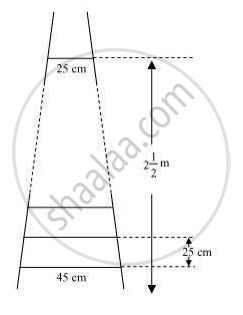# A ladder has rungs 25 cm apart. (See figure). The rungs decrease uniformly in length from 45 cm at the bottom to 25 cm at the top. If the top and bottom rungs are 2 1/2 m apart, what is the length of the wood required for the rungs? - Mathematics

A ladder has rungs 25 cm apart. (See figure). The rungs decrease uniformly in length from 45 cm at the bottom to 25 cm at the top. If the top and bottom rungs are 2 1/2 m apart, what is the length of the wood required for the rungs?

[Hint: number of rungs = 250/25 ]#### Solution

It is given that the rungs are 25 cm apart and the top and bottom rungs are 2 1/2 m apart

∴

Now, as the lengths of the rungs decrease uniformly, they will be in an A.P.

The length of the wood required for the rungs equals the sum of all the terms of this A.P.

First term, a = 45

Last term, l = 25

n = 11

Sn = n/2(a+1)

∴ S10 = 11/2(45+25) = 11/2 (70) = 385 cm

Therefore, the length of the wood required for the rungs is 385 cm

Concept: Sum of First n Terms of an AP
Is there an error in this question or solution?

#### APPEARS IN

NCERT Class 10 Maths
Chapter 5 Arithmetic Progressions
Exercise 5.4 | Q 3 | Page 115